Definitions of Square Dance Calls and Concepts

Index -->  Plus  |  A1  |  A2  |  C1  |  C2  |  C3A  |  C3B  |  C4  |  NOL  |
Definitions (Text Only) -->  Plus  |  A1  |  A2  |  C1  |  C2  |  C3A  |  C3B  |  C4  |  NOL  |
 Find call:

1/4 (or 3/4) Mix [C3A]
(author unknown)
 C3A:\$B8@8l(B

From a R-H Mini-Wave Box, a Diamond with the Centers in a R-H Mini-Wave, or other applicable formations. EN: 10
\$B1&\$N(B Mini-Wave \$B\$N(B Box, Center \$B\$,1&\$N(B Mini-Wave \$B\$N(B Diamond, \$B\$^\$?\$OB>\$NE,@Z\$J(B formation \$B\$+\$i!%(B JP: 10

1. Those who can Right Arm Turn 1/4 (or 3/4); EN: 20
\$B\$G\$-\$k?M\$,(B Right Arm Turn 1/4 (\$B\$^\$?\$O(B 3/4) \$B\$r\$7(B; JP: 20
2. those in a Line|Wave, Centers Cross Run; EN: 30
Line \$B\$+(B Wave \$B\$N?M\$,(B Centers Cross Run \$B\$r\$7\$^\$9!%(B; JP: 30
3. those in a Line|Wave, Centers Trade. EN: 40
Line \$B\$+(B Wave \$B\$N?M\$,(B Centers Trade \$B\$r\$7\$^\$9!%(B. JP: 40

Ends in a Line or Wave. EN: 50
Line \$B\$^\$?\$O(B wave \$B\$G=*\$o\$j\$^\$9!%(B JP: 50

1/4 (or 3/4) Mix is a 3-part call. EN: 60
1/4 (\$B\$^\$?\$O(B 3/4) Mix \$B\$O(B 3 \$B%Q!<%H\$N%3!<%k\$G\$9(B. JP: 60

R-H EN: 70
\$B1&\$N(B JP: 70
Mini-Wave Box \$B\$+\$i(B: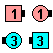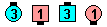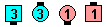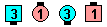1/4 Mix\$B\$NA0(B Right Arm Turn 1/4\$B\$N8e(B Centers Cross Run\$B\$N8e(B Centers Trade\$B\$N8e(B (\$B=*\$o\$j(B)
Diamond \$B\$+\$i(B: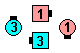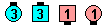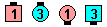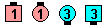1/4 Mix\$B\$NA0(B Centers Right Arm Turn 1/4\$B\$N8e(B Centers Cross Run\$B\$N8e(B Centers Trade\$B\$N8e(B (\$B=*\$o\$j(B)
\$BFCJL\$J(B formation \$B\$+\$i(B: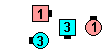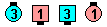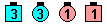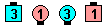1/4 Mix\$B\$NA0(B Those who can EN: 80 AddJapanese Right Arm Turn 1/4\$B\$N8e(B Centers Cross Run\$B\$N8e(B Centers Trade\$B\$N8e(B (\$B=*\$o\$j(B)

\$BCm
• 1/4 (or 3/4) Mix always starts with the Right hand! From Parallel L-H Waves, only the Centers can do the call. In our opinion, the caller should be explicit and say Centers 1/4 (or 3/4) MixEN: 90
1/4 (\$B\$^\$?\$O(B 3/4) Mix \$B\$O(B, \$B>o\$K(B\$B1&(B\$Bupdate JP: 90
• It is generally accepted that those starting the call must be in a Mini-Wave, not facing dancers. Most callers believe it is illegal to call 1/4 (or 3/4) Mix from a setup such as a Single Eight Chain Thru. EN: 100
\$B%3!<%k\$r;O\$a\$k?M\$O(B, \$B8~\$+\$\$9g\$C\$F\$\$\$k\$H\$3\$m\$+\$i\$G\$J\$/(B, Mini-Wave \$B\$G\$J\$1\$l\$P\$J\$i\$J\$\$(B, \$B\$H\$\$\$&\$3\$H\$O0lHLE*\$K1/4 (\$B\$^\$?\$O(B 3/4) Mix \$B\$,(B Single Eight Chain Thru \$B\$N\$h\$&\$J(B setup \$B\$+\$i%3!<%k\$5\$l\$k\$3\$H\$r\$*\$+\$7\$\$\$H;W\$C\$F\$\$\$^\$9!%(B JP: 100
• Left 1/4 (\$B\$^\$?\$O(B 3/4) Mix [C3A]:

\$B1&JP: 105

1/4 (or 3/4) Cross [C4] (Lee Kopman 1970):
Those who can Right Arm Turn 1/4 (or 3/4); those in a Line|Wave Centers Cross Run. EN: 30
\$B\$G\$-\$k?M\$,(B Right Arm Turn 1/4 (\$B\$^\$?\$O(B 3/4); Line \$B\$+(B Wave \$B\$N?M\$,(B Centers Cross Run. JP: 30
This is the same as 2/3 of a 1/4 (or 3/4) Mix. EN: 110
\$B\$3\$l\$O(B 2/3 of 1/4 (\$B\$^\$?\$O(B 3/4) Mix \$B\$G\$9!%(B JP: 110

Grand 1/4 (or 3/4) Mix [C3A]:
From R-H Columns (or other applicable formations). Those who can Right Arm Turn 1/4 (or 3/4); all Grand Mix. This is a 3-part call. EN: 160
\$B1&\$N(B Columns (\$B\$^\$?\$OB>\$NE,@Z\$J(B formation) \$B\$+\$i!%(B \$B\$G\$-\$k?M\$,(B Right Arm Turn 1/4 (\$B\$^\$?\$O(B 3/4) \$B\$r\$7\$F(B, \$BA40w\$G(B Grand Mix \$B\$r\$7\$^\$9!%(B 3 \$B%Q!<%H\$N%3!<%k\$G\$9!%(B JP: 160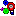Animation for 1/4 (or 3/4) MixChoreography for 1/4 (or 3/4) MixComments? Questions? Suggestions?

 C3A: